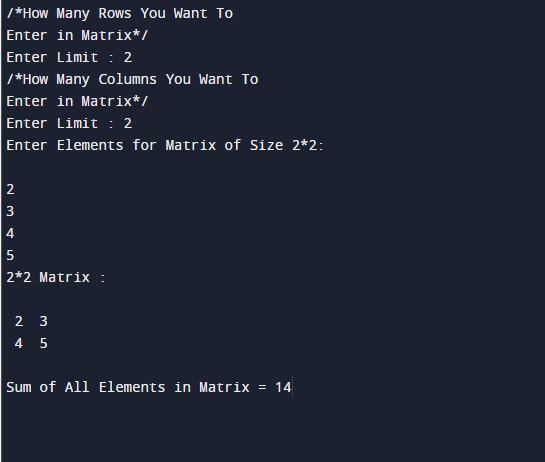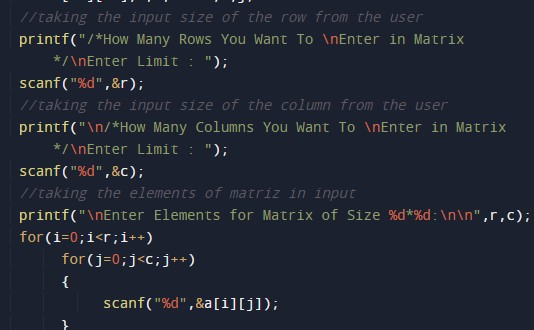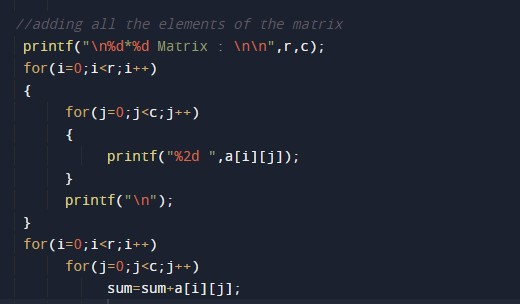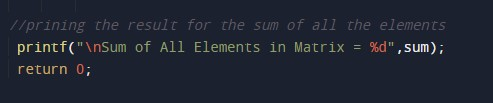C Program to Sum All Matrix Elements

In this tutorial you will learn about the C Program to Sum All Matrix Elements and its application with practical example.

C Program to Print Sum All Matrix Elements

In this tutorial, we will learn to create a C program that will Print Sum All Matrix Elements using C programming.

Prerequisites

Before starting with the tutorial we assume that you are best aware of the following C programming topics:

• Operators in C Programming.
• Basic Input and Output function in C Programming.
• Basic C programming.
• For loop in C programming.

Program to Print Sum of All Matrix Elements

In c programming, it is possible to take a numerical input for the size of the matrix and the elements of the matrix from the user and Print Sum of All Matrix Elements with the help of a very small amount of code. The C language has many types of header libraries which has supported function in them with the help of these files the programming is easy.

Algorithm:-

With the help of this program, we can Print Sum of All Matrix Elements.

Output:-In the above program, we have first initialized the required variable.

• a= it will hold the integer value.
• r= it will hold the integer value.
• c= it will hold the integer value.
• sum= it will hold the integer value.
• i= it will hold the integer value.
• j= it will hold the integer value.Taking the elements of the matrix from the user.Calculating the sun of all the elements of the matrix.Printing the sum for all the elements of the matrix.In this tutorial we have learn about the C Program to Sum All Matrix Elements and its application with practical example. I hope you will like this tutorial.